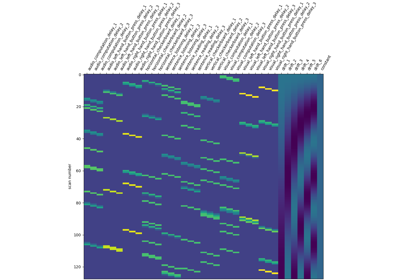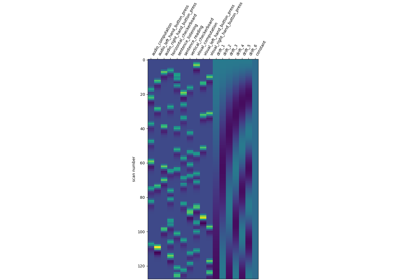Note

This page is a reference documentation. It only explains the function signature, and not how to use it. Please refer to the user guide for the big picture.

# nilearn.plotting.plot_contrast_matrix#

nilearn.plotting.plot_contrast_matrix(contrast_def, design_matrix, colorbar=False, ax=None, output_file=None)[source]#

Create plot for contrast definition.

Parameters:
contrast_def`str` or `numpy.ndarray` of shape (n_col), or `list` of `str`, or `numpy.ndarray` of shape (n_col)

where `n_col` is the number of columns of the design matrix, (one array per run). If only one array is provided when there are several runs, it will be assumed that the same contrast is desired for all runs. The string can be a formula compatible with `pandas.DataFrame.eval`. Basically one can use the name of the conditions as they appear in the design matrix of the fitted model combined with operators +- and combined with numbers with operators +-*/.

design_matrix`pandas.DataFrame`

Design matrix to use.

colorbar`bool`, optional

If True, display a colorbar on the right of the plots. Default=False.

ax`matplotlib.axes.Axes`, optional

Axis on which to plot the figure. If None, a new figure will be created.

output_file`str`, or None, optional

The name of an image file to export the plot to. Valid extensions are .png, .pdf, .svg. If output_file is not None, the plot is saved to a file, and the display is closed.

Returns:
ax`matplotlib.axes.Axes`

Figure object.

## Examples using `nilearn.plotting.plot_contrast_matrix`#Intro to GLM Analysis: a single-session, single-subject fMRI dataset

Intro to GLM Analysis: a single-session, single-subject fMRI datasetAnalysis of an fMRI dataset with a Finite Impule Response (FIR) model

Analysis of an fMRI dataset with a Finite Impule Response (FIR) modelFirst level analysis of a complete BIDS dataset from openneuro

First level analysis of a complete BIDS dataset from openneuroUnderstanding parameters of the first-level model

Understanding parameters of the first-level model